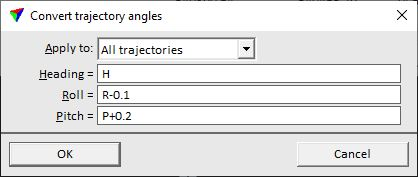# TerraPhoto User Guide

 Navigation: Tool Reference > Manage Trajectories > Tools pulldown menu Convert angles

Convert angles

Convert angles command lets you apply a mathematical equation to the orientation angles heading, pitch, and roll of each trajectory position. The current angle value can be accessed by using constants H (heading), R (roll), and P (pitch). Thus, the command can also be used to exchange angle values.

To convert angles of trajectory positions:

1. (Optional) Select trajectory file(s) for which to manipulate angles.

2. Select Convert angles command from the Tools pulldown menu.

This opens the Convert trajectory angles dialog:3. Define equations an click OK.

This computes the new values for the orientation angles. The modification is saved to the trajectory binary files in the active trajectory directory. An information dialog shows the number of effected trajectories.

SETTING

EFFECT

Apply to

Trajectories for which the computation of new angles is applied:

All trajectories - all trajectories in the list.

Selected only - selected trajectories only.